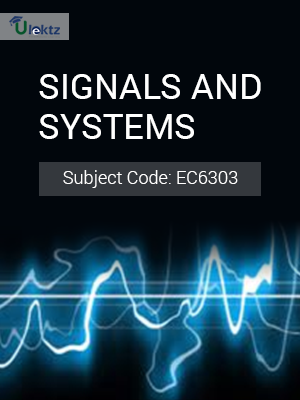•My WalletMy Order
•My Profile
•My Connections
•My Books
•My Videos
•My Tests
•My Calender
•My Messages
•My Shopping Cart
•My Orders
•Account Settings
•Help

# Book Details# Signals and Systems

 Course Code : EC6303 Author : uLektz University : Anna University, Tamil Nadu Regulation : 2013 Categories : Electronics & Communication Format :ePUB3 (DRM Protected) Type : eBook

FREE

Description :Signals and Systems of EC6303 covers the latest syllabus prescribed by Anna University, Tamil Nadu for regulation 2013. Author: uLektz, Published by uLektz Learning Solutions Private Limited.

Note : No printed book. Only ebook. Access eBook using uLektz apps for Android, iOS and Windows Desktop PC.

##### Topics
###### UNIT I CLASSIFICATION OF SIGNALS AND SYSTEMS

1.1 Continuous time signals (CT signals) - Discrete time signals (DT signals)

1.2 Periodic & Aperiodic signals

1.3 Energy & Power signals

1.4 Classification of CT and DT signals(Step, Ramp, Pulse, Impulse, Sinusoidal, Exponential)

1.5 CT systems and DT systems

1.6 Classification of systems –Static & Dynamic, Linear & Nonlinear, Time-variant & Time-invariant, Causal & Noncausal, Stable & Unstable.

###### UNIT II ANALYSIS OF CONTINUOUS TIME SIGNALS

2.1 Fourier series Analysis

2.2 Properties of continuous time fourier transform and laplace transform

2.3 Common Pairs of CT-FT and CT-Laplace Transform

2.4 Continuous Time – Laplace Transform Analysis

2.5 Continuous Time – Fourier Transform Analysis

###### UNIT III LINEAR TIME INVARIANT- CONTINUOUS TIME SYSTEMS

3.1 Convolution Integral – Time Domain

3.2 Convolution Integral – Laplace Transform

3.3 Convolution Integral – Fourier Transform

3.4 Differential Equation –Without Initial condition (Using Laplace, Fourier Transform)

3.5 Differential Equation – With Initial condition (Using Laplace, Fourier Transform)

3.6 Block Diagram Representation

###### UNIT IV ANALYSIS OF DISCRETE TIME SIGNALS

4.1 Baseband Sampling

4.2 properties of discrete time fourier transform and z-transform

4.3 DTFT and inverse DTFT, Properties

4.4 Z-Transform, Properties, Convolution

###### UNIT V LINEAR TIME INVARIANT-DISCRETE TIME SYSTEMS

5.1 Difference Equations

5.2 Block diagram representation, IIR Realization, Types of FIR Realization:

5.3 Impulse response

5.4 Convolution sum

5.5 Discrete Fourier and Z Transform Analysis of Recursive & Non-Recursive systems, Recursive And Non-Recursive Discrete Time System

### Related Books